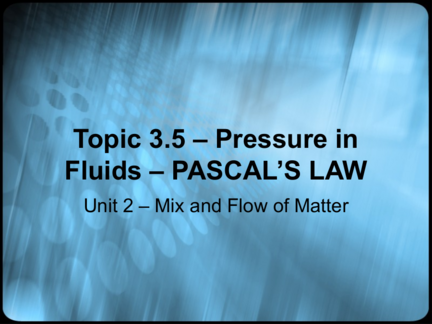# Pressure in Fluids – PASCAL’S LAWContributed by:Pressure, Formula, Pressure and depth, Pascal's law, Hydraulic devices
1. Topic 3.5 – Pressure in
Fluids – PASCAL’S LAW
Unit 2 – Mix and Flow of Matter
2. UNITS
– Name me as many units as you can think
of…
– GO!!!!
3. Pressure
• Pressure is the amount of force applied
to a given area.
– Measured in pascals (Pa)
• A pascal equals the force of 1 N (newton) over
an area of 1 m2
• The MORE force you can apply to an
area, the GREATER the pressure
4. Formula
• The formula for calculating pressure is:
P=F
A
P = pressure (Pa)
F = force (N)
A = area (m2)
5. Example
• You have a force of 10 N on an area of 2 m2. What
would the pressure be?
Area = 2 m2
Force = 10 N
Pressure = ?
P = 10 N
2 m2
P = 5 Pa
6. Kilopascals
• A pascal is a VERY SMALL amount of
pressure.
– Most scientists will measure pressure in
kilopascals (kPa)
• 1 kPa = 1000 Pa
• A ballet dancer standing on the toes of
ONE foot exerts 2500 kPa of pressure on
the floor.
– How many pascals is this?
7. Pressure and Depth
• Water in a tin can is
exerting pressure on all
the walls of the tin can
• If we were to punch
TWO holes in this tin
can, how would the
water come out?
8. Tin can experiment
• The water is coming out almost in the exact
same way.
– Why is this?
• The weight of the water in the upper part of
the tin can is pressed down on the water in
the lower part.
– The more water above the hole, the more
pressure.
9. Pascal’s Law
• Meet Pascal --------
– Handsome man eh?
• Pascal developed a law
to explain how pressure
is equal in all directions
in fluids
10. • Pascal’s Law states that an enclosed
fluid transmits pressure EQUALLY in all
directions.
• Let’s look at some examples to figure
out what the heck this handsome man
(haha) is trying to tell us 
11. Hydraulic Devices
• Hydraulic systems use a liquid as the
enclosed fluid
– Just like we saw in Bill Nye with the cars.
12. Car lifts
• In the lift we just saw, the output force is
16 TIMES greater than the input force.
• A benefit of this type of system is it can
multiply force.
14. Pneumatic Systems
• Pneumatic systems use air to do
– Examples of this would be
• Dentist drills, jack hammers, paint sprayers and
air brakes on trucks
• These cost less and are more safe than
hydraulic systems
15. Example
• Study the diagram below. If the input
force is 100N, what is the output
force?
5m2 50m2
16. Maintaining Pressure
• For a hydraulic and pneumatic system
to function properly, the entire system
must be SEALED
– The smallest hole or leak causes the
system to fail.